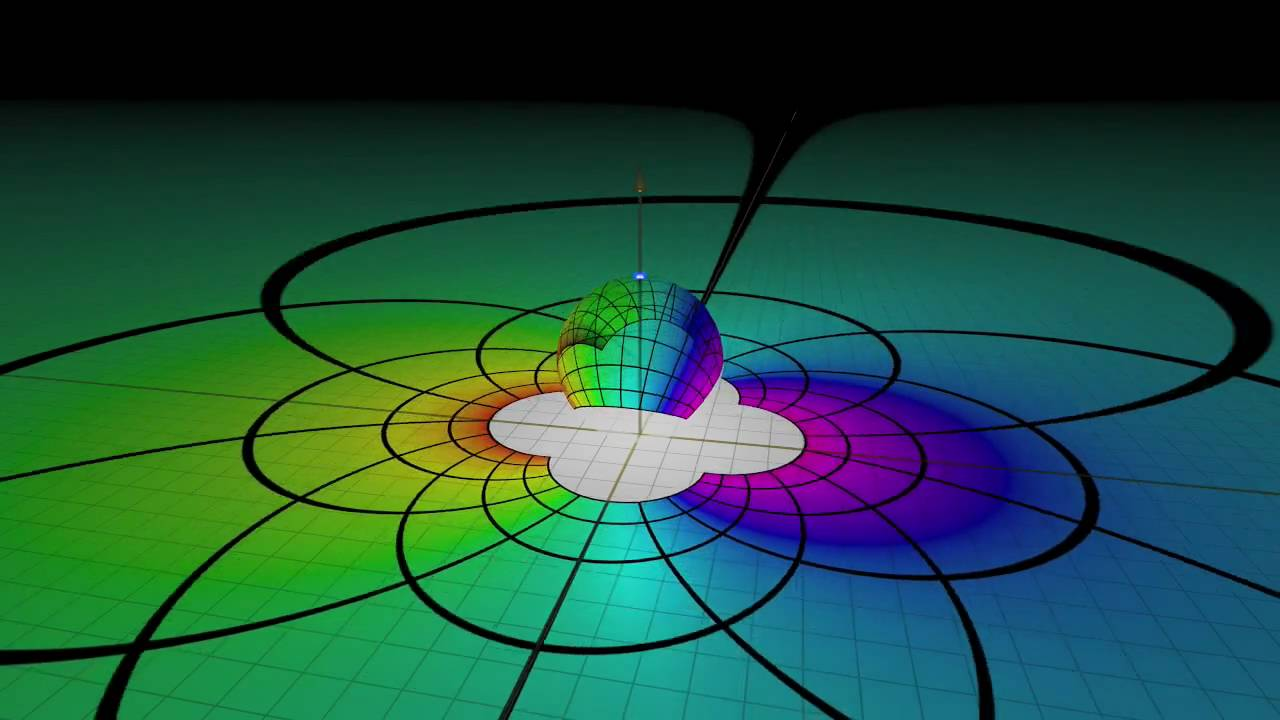# Does an stereographical projection of a physical plane have any physical meaning?

Mathematically, an arbitrary 2D plane can be mapped onto a sphere by stereographical projection. Each line on the plane is equivalent to each line on the sphere. If the sphere rotates under the $SO(3)$ group, the plane is transformed under the Möbius group.If the plane has some physical meanings (coordinate plane, phase plane), then does its stereographical projection have some physical meanings too? What happens if the plane is multi-dimensional, and the group is different than $SO(n)$? Would it be just math, not physics anymore? How about the group $SU(3)\times SU(2)\times U(1)$ in particle physics?

Since it is the Riemann sphere in complex analysis, complex dynamics might have an answer this, but on the first skim it seems to be more about fractals, not stereographical projection.

Some of my related questions:
Is there a relation between phase plane and complex plane?
Roadmap to understand the link between harmonic analysis and Riemann sphere?

• This post (v3) seems to be a list question. Riemann surfaces are e.g. used in string theory; the Bloch sphere is a Riemann sphere; etc. – Qmechanic Oct 14 '17 at 8:22
• Is list question accepted? – Ooker Oct 14 '17 at 8:24
• The Wikipedia page on the Riemann sphere lists some applications in physics. – diracula Oct 14 '17 at 12:30